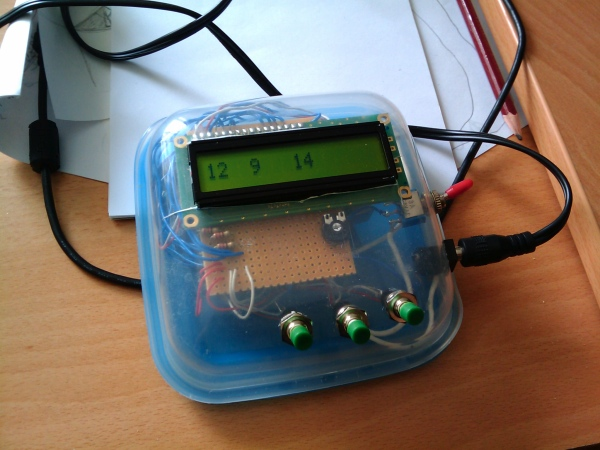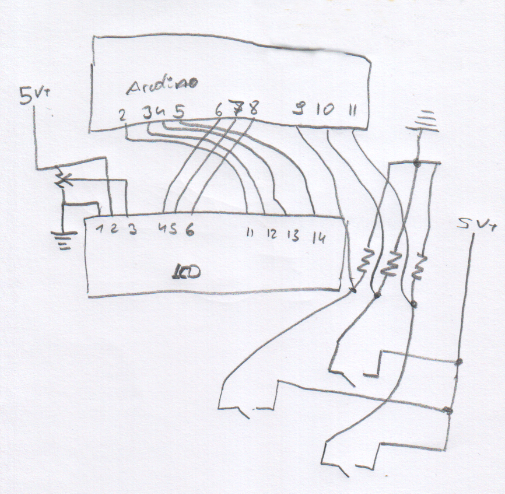## Adrduino Counter with lcd Display

I made a small device that has 3 independent counters which are shown on a lcd-display. The device uses an arduino and has 3 buttons on the front that are debounced in software and used to increment a variable. The variables are printed on a 1x16 char lcd-display.

the red switch on the side is a power-switch.i really like the "frankenstein"-look of the eclosure :-)

i also made some sort of a "schematic". scroll down to see the arduino sketch i'm usingarduino sketch:

``````#include <LiquidCrystal.h>

LiquidCrystal lcd(6, 7, 8, 2, 3, 4, 5);

const int pinA = 9;
const int pinB = 10;
const int pinC = 11;

int lastA = LOW;
int lastB = LOW;
int lastC = LOW;

int stateA;
int stateB;
int stateC;

const long DELAY = 100;

long lastATime = 0;
long lastBTime = 0;
long lastCTime = 0;

void setup() {
// Print a message to the LCD.
lcd.clear();
lcd.setCursor(0,0);
lcd.print("hello, w");
lcd.setCursor( 0, 1);
lcd.print("orld!");

delay(1000);
lcd.clear();

pinMode( pinA, INPUT );
pinMode( pinB, INPUT );
pinMode( pinC, INPUT );
pinMode( 13, OUTPUT );

printNumbers(0, 0, 0);
}

int a, b, c = 0;
boolean changed = false;

void loop() {
changed = false;

digitalWrite( 13, HIGH );
} else {
digitalWrite( 13, LOW );
}

a++;
changed = true;
}

b++;
changed = true;
}

c++;
changed = true;
}

if (changed) printNumbers( a, b, c );

}

void printNumbers( int a, int b, int c ) {
lcd.setCursor(0,0);
lcd.print(a);
lcd.setCursor(4,0);
lcd.print(b);
lcd.setCursor(0, 1);
lcd.print(c);
}

int trippleRead( int pin ) {

int r1 = digitalRead( pin );
delay(10);
int r2 = digitalRead( pin );
delay(10);
int r3 = digitalRead( pin );

return ( r1 == HIGH && r2 == HIGH && r3 == HIGH ? HIGH : LOW );

}
``````

Leave a response

1. man, that tripple read is a neat way to debounce a switch!

if you dont mind, i think i'll be using your code for my projects!

2. Hi Please I need your help I am working with car parking system project using Arduino. In my project I use seven segment to display the number of parking free or number of cars. I want to add the LCD to display the number of free parking after each car. I have the complete code but i want to add the LCD code.

3. Hey, can I do the same using 2x16 LCD Module ?

4. //for lcd 16x2 i2c

# include <LiquidCrystal_I2C.h>

LiquidCrystal_I2C lcd(0x3F, 2, 1, 0, 4, 5, 6, 7, 3, POSITIVE);

const int pinA = 10; const int pinB = 11; const int pinC = 12; const int pinD = 9;

int lastA = LOW; int lastB = LOW; int lastC = LOW; int lastD = LOW;

void setup() { // Print a message to the LCD. lcd.begin(16, 2); lcd.setCursor(2, 0); lcd.print("HELLO"); lcd.setCursor( 8, 0); lcd.print("WORLD");

delay(1000); lcd.clear();

pinMode( pinA, INPUT ); pinMode( pinB, INPUT ); pinMode( pinC, INPUT ); pinMode( pinD, INPUT ); pinMode( 13, OUTPUT );

printNumbers(0, 0, 0, 0); }

int a, b, c, d = 0; boolean changed = false;

void loop() { changed = false;

if ( readA == HIGH || readB == HIGH || readC == HIGH || readD == HIGH) { digitalWrite( 13, LOW ); } else { digitalWrite( 13, HIGH ); }

if ( readA != lastA && readA == HIGH ) { a++; changed = true; }

if ( readB != lastB && readB == HIGH ) { b++; changed = true; }

if ( readC != lastC && readC == HIGH ) { c++; changed = true; } if (readD != lastD && readD == HIGH ) { d++; changed = true; }

if (changed) printNumbers( a, b, c , d);

}

void printNumbers( int a, int b, int c , int d) { lcd.setCursor(1, 0); lcd.print(a); lcd.setCursor(5, 0); lcd.print(b); lcd.setCursor(9, 0); lcd.print(c); lcd.setCursor(13, 0 ); lcd.print(d); }

int trippleRead( int pin ) {

int r1 = digitalRead( pin ); delay(10); int r2 = digitalRead( pin ); delay(10); int r3 = digitalRead( pin ); delay(10); int r4 = digitalRead( pin ); delay(10);

return ( r1 == HIGH && r2 == HIGH && r3 == HIGH && r4 == HIGH ? HIGH : LOW );

}

5. I am rrying to make this but with a small ssd1306 i2c display. Sadly only getting a screen of stars just now but I'm working on it.